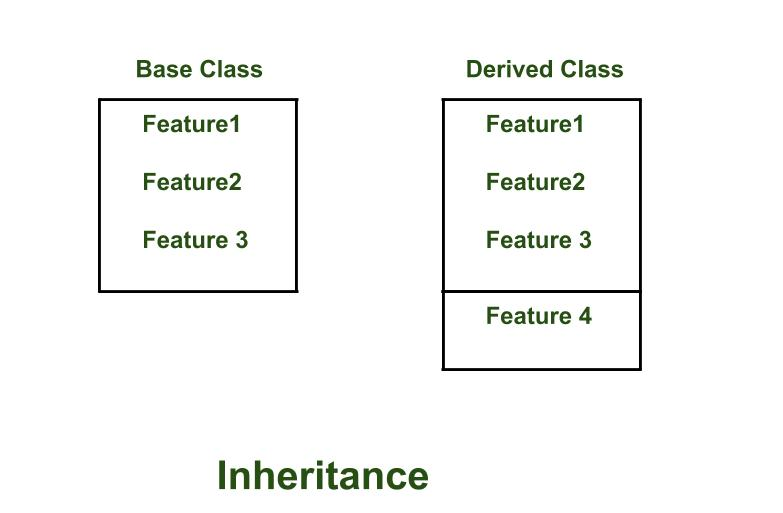# Python3 Intermediate Level Topics

After going through the basics of python, you would be interested to know more about further and bit more advance topics of the Python3 programming language.
Please remember that Python completely works on indentation and it is advised to practice it a bit by running some programs. Use the tab key to provide indentation to your code.

1. Classes
Just like every other Object Oriented Programming language Python supports classes. Let’s look at some points on Python classes.

• Classes are created by keyword class.
• Attributes are the variables that belong to class.
• Attributes are always public and can be accessed using dot (.) operator. Eg.: Myclass.Myattribute

A sample E.g for classes:

 `# creates a class named MyClass ` `class` `MyClass:   ` `        ``# assign the values to the MyClass attributes ` `        ``number ``=` `0`        `        ``name ``=` `"noname"` ` `  `def` `Main(): ` `        ``# Creating an object of the MyClass.  ` `        ``# Here, 'me' is the object ` `        ``me ``=` `MyClass()  ` ` `  `        ``# Accessing the attributes of MyClass ` `        ``# using the dot(.) operator    ` `        ``me.number ``=` `1337`     `        ``me.name ``=` `"Harssh"` ` `  `        ``# str is an build-in function that  ` `        ``# creates an string ` `        ``print``(me.name ``+` `" "` `+` `str``(me.number)) ` `    `  `# telling python that there is main in the program. ` `if` `__name__``=``=``'__main__'``:   ` `        ``Main() `

Output :

```Harssh 1337
```
2. Methods
Method is a bunch of code that is intended to perform a particular task in your Python’s code.

• Function that belongs to a class is called an Method.
• All methods require ‘self’ parameter. If you have coded in other OOP language you can think of ‘self’ as the ‘this’ keyword which is used for the current object. It unhides the current instance variable.’self’ mostly work like ‘this’.
• ‘def’ keyword is used to create a new method.

 `# A Python program to demonstrate working of class ` `# methods ` ` `  `class` `Vector2D: ` `        ``x ``=` `0.0` `        ``y ``=` `0.0` ` `  `        ``# Creating a method named Set ` `        ``def` `Set``(``self``, x, y):      ` `                ``self``.x ``=` `x ` `                ``self``.y ``=` `y ` ` `  `def` `Main(): ` `        ``# vec is an object of class Vector2D ` `        ``vec ``=` `Vector2D()    ` `        `  `        ``# Passing values to the function Set ` `        ``# by using dot(.) operator. ` `        ``vec.``Set``(``5``, ``6``)        ` `        ``print``(``"X: "` `+` `str``(vec.x) ``+` `", Y: "` `+` `str``(vec.y)) ` ` `  `if` `__name__``=``=``'__main__'``: ` `        ``Main() `

Output :

`X: 5, Y: 6`
3. Inheritance
Inheritance is defined as a way in which a particular class inherits features from its base class.Base class is also knows as ‘Superclass’ and the class which inherits from the Superclass is knows as ‘Subclass’As shown in the figure the Derived class can inherit features from its base class, also it can define its own features too.

 `# Syntax for inheritance ` ` `  `class` `derived``-``classname(superclass``-``name) `

 `# A Python program to demonstrate working of inheritance ` `class` `Pet: ` `        ``#__init__ is an constructor in Python ` `        ``def` `__init__(``self``, name, age):      ` `                ``self``.name ``=` `name ` `                ``self``.age ``=` `age ` ` `  `# Class Cat inheriting from the class Pet ` `class` `Cat(Pet):          ` `        ``def` `__init__(``self``, name, age): ` `                ``# calling the super-class function __init__  ` `                ``# using the super() function ` `                ``super``().__init__(name, age)  ` ` `  `def` `Main(): ` `        ``thePet ``=` `Pet(``"Pet"``, ``1``) ` `        ``jess ``=` `Cat(``"Jess"``, ``3``) ` `         `  `        ``# isinstance() function to check whether a class is  ` `        ``# inherited from another class ` `        ``print``(``"Is jess a cat? "` `+``str``(``isinstance``(jess, Cat))) ` `        ``print``(``"Is jess a pet? "` `+``str``(``isinstance``(jess, Pet))) ` `        ``print``(``"Is the pet a cat? "``+``str``(``isinstance``(thePet, Cat))) ` `        ``print``(``"Is thePet a Pet? "` `+``str``(``isinstance``(thePet, Pet))) ` `        ``print``(jess.name) ` ` `  `if` `__name__``=``=``'__main__'``: ` `        ``Main() `

Output :

```Is jess a cat? True
Is jess a pet? True
Is the pet a cat? False
Is thePet a Pet? True
Jess
```
4. Iterators
Iterators are objects that can be iterated upon.

• Python uses the __iter__() method to return an iterator object of the class.
• The iterator object then uses the __next__() method to get the next item.
• for loops stops when StopIteration Exception is raised.

 `# This program will reverse the string that is passed ` `# to it from the main function ` `class` `Reverse: ` `    ``def` `__init__(``self``, data): ` `        ``self``.data ``=` `data ` `        ``self``.index ``=` `len``(data)         ` ` `  `    ``def` `__iter__(``self``): ` `        ``return` `self` `     `  `    ``def` `__next__(``self``): ` `        ``if` `self``.index ``=``=` `0``: ` `            ``raise` `StopIteration     ` `        ``self``.index``-``=` `1` `        ``return` `self``.data[``self``.index] ` ` `  `def` `Main(): ` `    ``rev ``=` `Reverse(``'Drapsicle'``) ` `    ``for` `char ``in` `rev: ` `        ``print``(char) ` ` `  `if` `__name__``=``=``'__main__'``: ` `    ``Main() `

Output :

```e
l
c
i
s
p
a
r
D
```
5. Generators
• Another way of creating iterators.
• Uses a function rather than a separate class
• Generates the background code for the next() and iter() methods
• Uses a special statement called yield which saves the state of the generator and set a resume point for when next() is called again.

 `# A Python program to demonstrate working of Generators ` `def` `Reverse(data): ` `    ``# this is like counting from 100 to 1 by taking one(-1)  ` `    ``# step backward. ` `    ``for` `index ``in` `range``(``len``(data)``-``1``, ``-``1``, ``-``1``): ` `        ``yield` `data[index] ` ` `  `def` `Main(): ` `    ``rev ``=` `Reverse(``'Harssh'``) ` `    ``for` `char ``in` `rev: ` `        ``print``(char) ` `    ``data ``=``'Harssh'` `    ``print``(``list``(data[i] ``for` `i ``in` `range``(``len``(data)``-``1``, ``-``1``, ``-``1``))) ` ` `  `if` `__name__``=``=``"__main__"``: ` `    ``Main() `

Output :

```h
s
s
r
a
H
['h', 's', 's', 'r', 'a', 'H']
```

My Personal Notes arrow_drop_up

Improved By : Akanksha_Rai

Article Tags :

27

Please write to us at contribute@geeksforgeeks.org to report any issue with the above content.